# Mixolumia

Entrancing musical block-clearing puzzler · By davemakes

# Color Palettes Sticky

A topic by davemakes created 82 days ago Views: 1,152 Replies: 37
Viewing posts 1 to 24
Developer (4 edits) (+1)

Share your color palettes here if you want! Include a screenshot and all the info to paste into the color palette ini file.

Mixolumia comes with a bunch of color palettes, but you can make your own, too! Here's a template you can download and edit to help visualize your colors: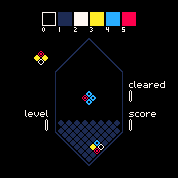You will need to edit the following file...

```WIN: %LOCALAPPDATA%/Mixolumia/user_palettes.ini
MAC: ~/Library/Application Support/com.davemakes.mixolumia/user_palettes.ini```

You may need to run Mixolumia once to generate this file.

There are six hexadecimal colors in a Mixolumia palette. The format for the .ini file goes like this:

```
name=Pico Dark
0=000000
1=1d2b53
2=fff7f2
3=ffec27
5=ff004d


name=Pico Light
0=fff1e8
1=f0d4d3
2=1d2b53
3=ffa300
5=ff004d


name=Grim Roguelike
author=Noé Charron @celechii
0=3c0902
1=290402
2=fab7af
3=d2001e
5=fab7af```

Make sure you number each palette sequentially, no skipping or repeating numbers or else your palette might not load correctly.

The colors correspond to these game parts:
0: Background
1: Game Board
2: Empty Block and Text
3: Filled Block and Selected Text
4: Hole Block
5: Target Block

Pro Tip: Colors 0 and 1 should have pretty low contrast with each other or else it will be hard to see the pieces when things get wild.

Make sure that each entry has a unique number, and there are no breaks between numbers. Show me your color palettes, and maybe I'll include them in future releases!

(+2)

For when you need to hack your way into the mainframe

name="Hackerman"
author="@matvosty"
0="000000"
1="242424"
2="97ff52"
3="acff69"
4="46b516"
5="0bba1a"

a pallette based on Sevipers!

name=:3~<
author="MattTheSpratt"
0=000000
1=262646
2=4b4b78
3=7974e1
4=fd4760
5=ffda60

I've put together a couple that I think are pretty nice!

name=Heart of the Forest
author=Heather Flowers @HTHRFLWRS
0=3d200f
1=543d22
2=00b566
3=ff7c31
4=9cbfb3
5=c4ff79

name=Meatolumia
author=Heather Flowers @HTHRFLWRS
0=2d0604
1=400b01
2=fbeba4
3=fcef01
4=f78003
5=ef3a00

Developer

Heather these are so lovely!

(2 edits) (+4)

This is so fun! I made one based on a game of mine. If anyone know what the second one is referencing you win a prize (the prize is me saying "oh cool you figured it out!"). NOTE: For me using the itch app on Windows 7, the folder was here - C:\Users\USERNAME\AppData\Roaming\itch\apps\mixolumia\data

name="Even the Ocean"
author="@even_kei"
0="171c23"
1="35392f"
3="ff5a80"
4="33efa6"
5="7d52e9"

name="Eliminating the Grid"
author="@even_kei h/t Tsugumo"
0="002255"
1="083b8c"
2="26befa"
3="00ff55"
4="007774"
5="f70c27"

Developer (1 edit) (+1)

So there's two folders, the actual game resources folder and the user folder. You can edit both, but if you change stuff in the game's folder it may cause conflicts or get overwritten when updates come. So I suggest you stick to editing:

`C:\Users\USERNAME\AppData\Local\Mixolumia\user_palettes.ini`

Ah, so the reason I used the other folder was that for me there is no Mixolumia folder in that location! So I was trying to find a different one

I know the second one is a reference to something, so the name is probably important, but "Get Rid of the Grid" is a good contender.

(1 edit) (+8)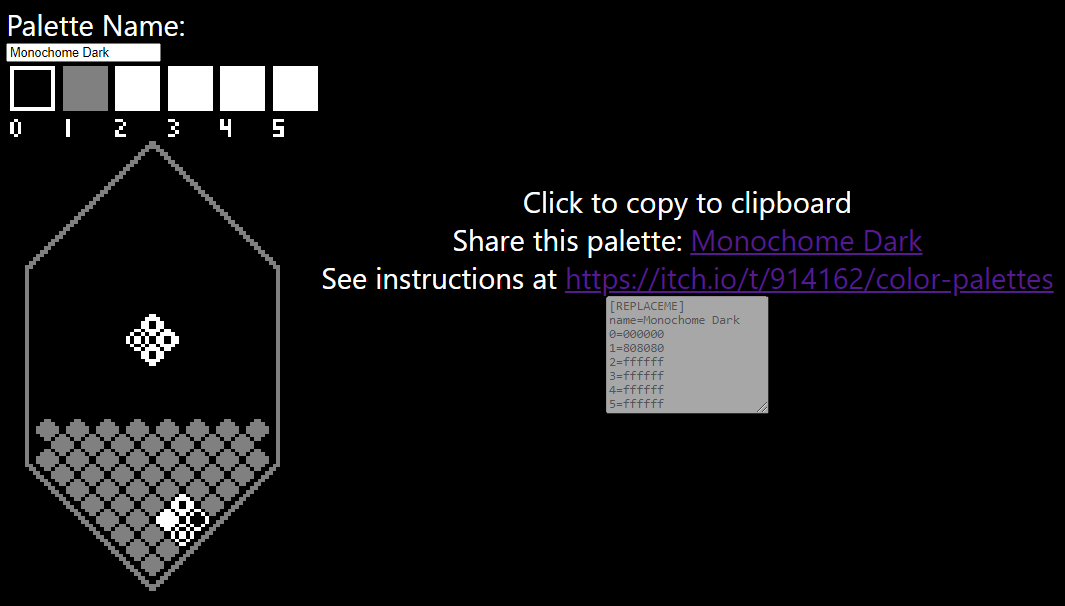Hello! I made a little web app to generate and share color palettes. I've still got a bit of enhancements I want to make but it's functional enough that it should be useful to this channel. Try it out : D

https://mixolumia-palette-picker.netlify.app/?0=000000&1=808080&2=ffffff&3=ffffff&4=ffffff&5=ffffff&name=Monochome%20Dark

I'm using React and hosting on Netlify and the code is OSS here: https://github.com/FLGMwt/mixolumia-palette-picker

Developer(+2)

THIS IS AMAZING!

(1 edit) (+4)

Awesome tool! Here are the two I use:

name=Waffle Cone
author=Calamari
0=957b50
1=382b16
2=fbf96c
3=ffffff
4=6cf18f
5=fb77e9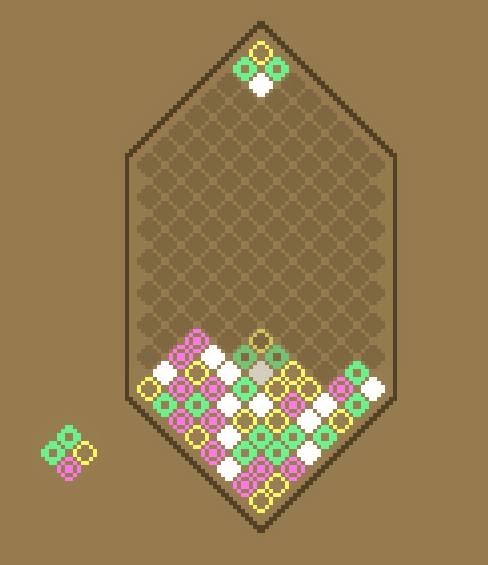name=Power Chord
author=Calamari
0=2e0232
1=5b164b
2=e4a942
3=6ee4a9
4=52bdd9
5=ef2d27

Developer(+1)

Power Chord is amazing!! it's like radioactive punk rock slime injected into my retinas and I love it

The best description of a color palette I've ever heard haha. Glad you like it!

i love waffle cone!! <3

Thanks~

(7 edits) (+2)

Amazing tool! Thanks!

Here is one I created based on the color palette for "The Legend of Zelda" on the NES: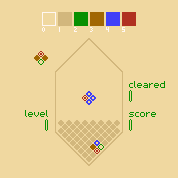name="The Hero"
author="sofamire"
0=f0d8a0
1=d2b77d
2=089000
3=a16703
4=4040f8
5=b03020

Here is one based on darker base colors, and then purple, orange and white: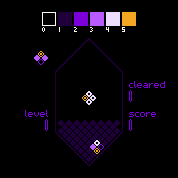name=Nightmare
author="sofamire"
0=000000
1=1f003b
2=7c00da
3=b75bff
4=efddff
5=f5a623

Here is one based off of Cherry Blossom/Sakura trees. The ripple effect looks really good as a kind of "water" in this one: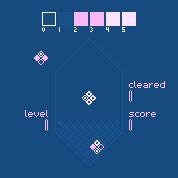name="Sakura"
author="sofamire"
0=164b7f
1=19528b
2=fcb8f4
3=fcb8f4
4=fce4ff
5=fce4ff

(2 edits) (+1)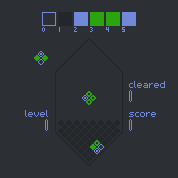a palette based on my own usual set of colors which most of them are taken from Discord's colors

name=Omega
author=@Omegacreeper
0=2C2F33
1=23272A
2=7289DA
3=2aa50f
4=2aa50f
5=7289DA

This is awesome!

(1 edit) (+2)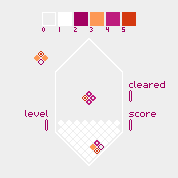use these colors to make mixolumia extra lesbian :^)

name=Sapphic
author=reeseovine
0=eeeeee
1=ffffff
2=a20161
3=fd9855
4=be1c7d
5=d4380f

Developer(+1)

omg i love this

Here's a light non binary color palette

name="Light nb"

author="stanato"

0="ffffff"
1="FFFEC7"
2="434343"
3="E7ACFF"
4="434343"
5="FFF571"

(1 edit) (+2)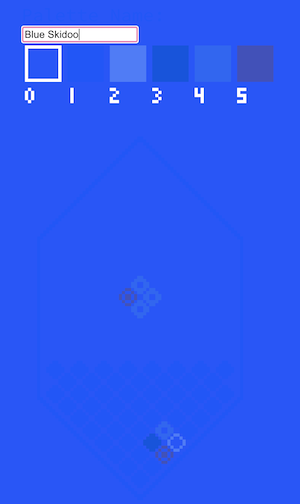Blue Skidoo

name=Blue Skidoo
0=244fff
1=1a4fff
2=4779fd
3=024fe2
4=2662f8
5=414eb

'Everybody Falls' Mixolumia x Fall Guys Palette

[REPLACEME]
name=Everybody Falls
0=f9cb16
2=d52020
3=ffffff
4=e3317e
5=282428

'Time Vortex' Colour Palette Inspired by Doctor Who and the 13th Doctor's Rainbow Jumper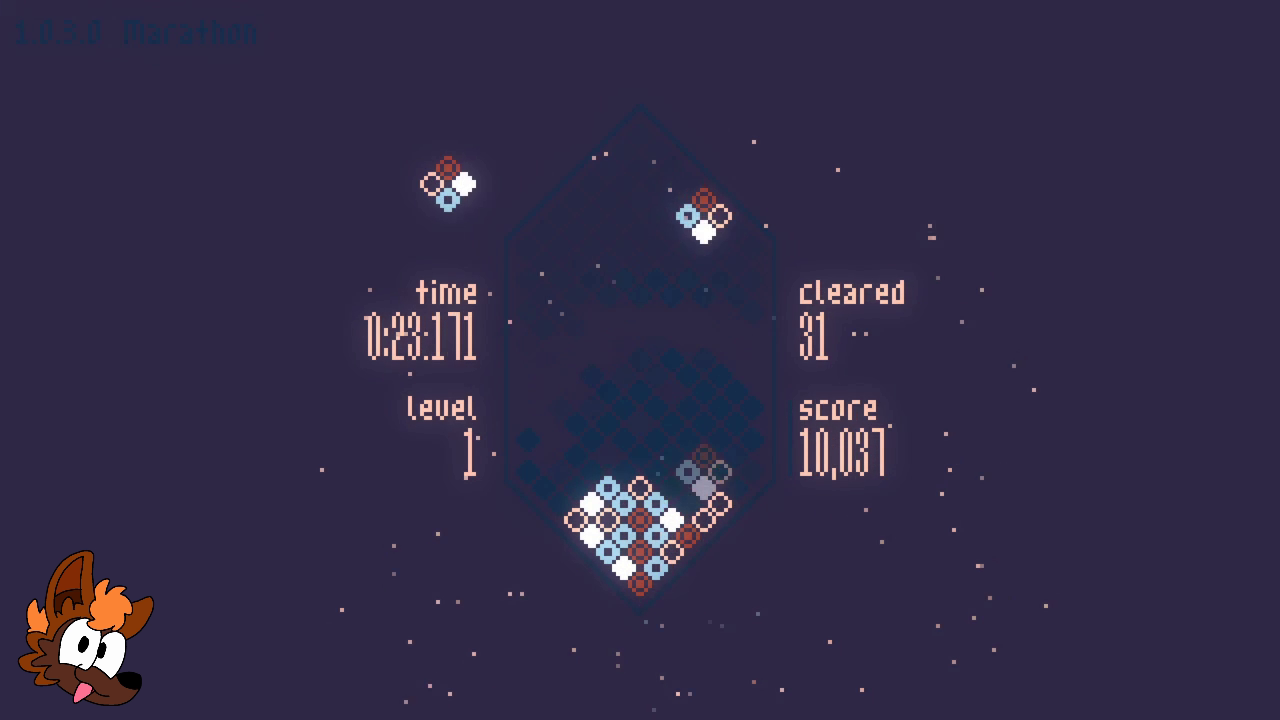[REPLACEME]

name=Time Vortex

0=252037
1=0f253d
2=eeb49d
3=ffffff
4=8ab4c7
5=8a3325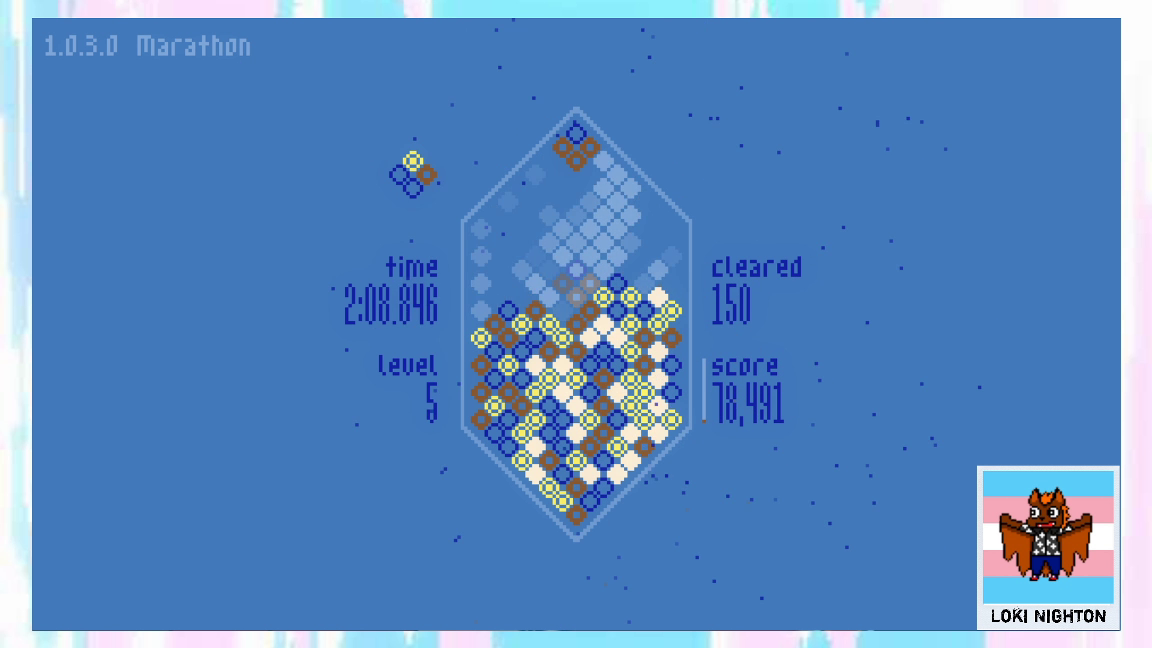[REPLACEME]
name=Vault Boy
0=447ab9
1=82a7d6
2=131da3
3=feeed4
4=8b572a
5=fef265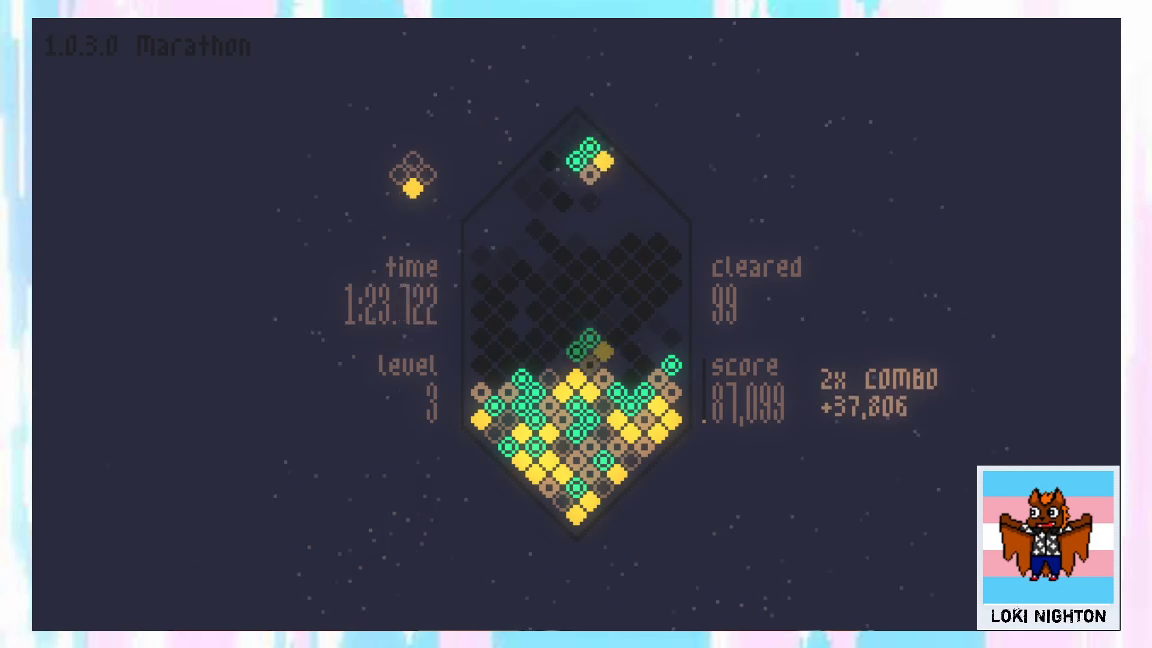[REPLACEME]
name=Ash Heap
0=1d1e2a
1=141414
2=634c44
3=d9af2f
4=8c6a50
5=1aff80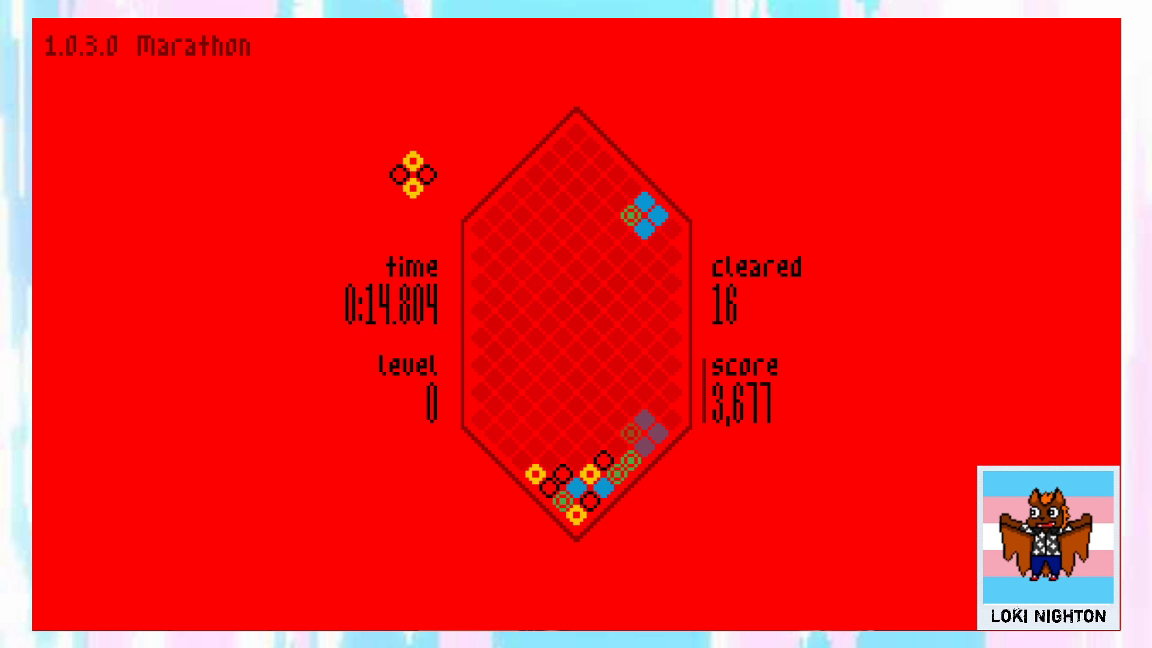[REPLACEME]
name=Mariolumia
0=fe0002
1=6a0400
2=000000
3=049cd8
4=fbd000
5=43b047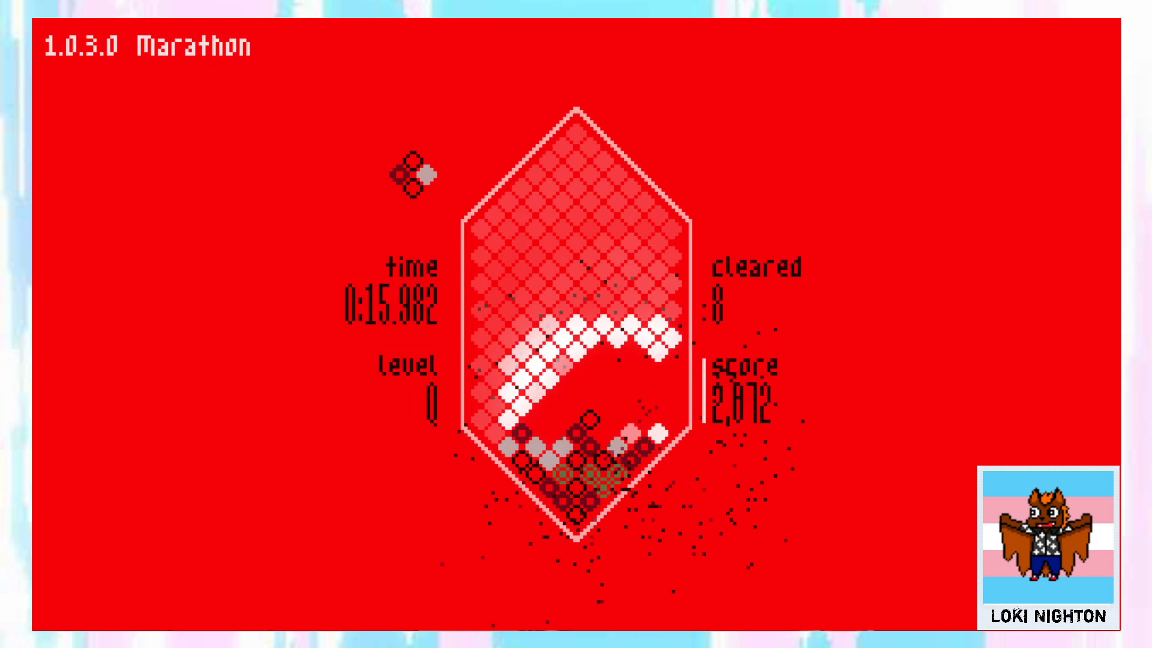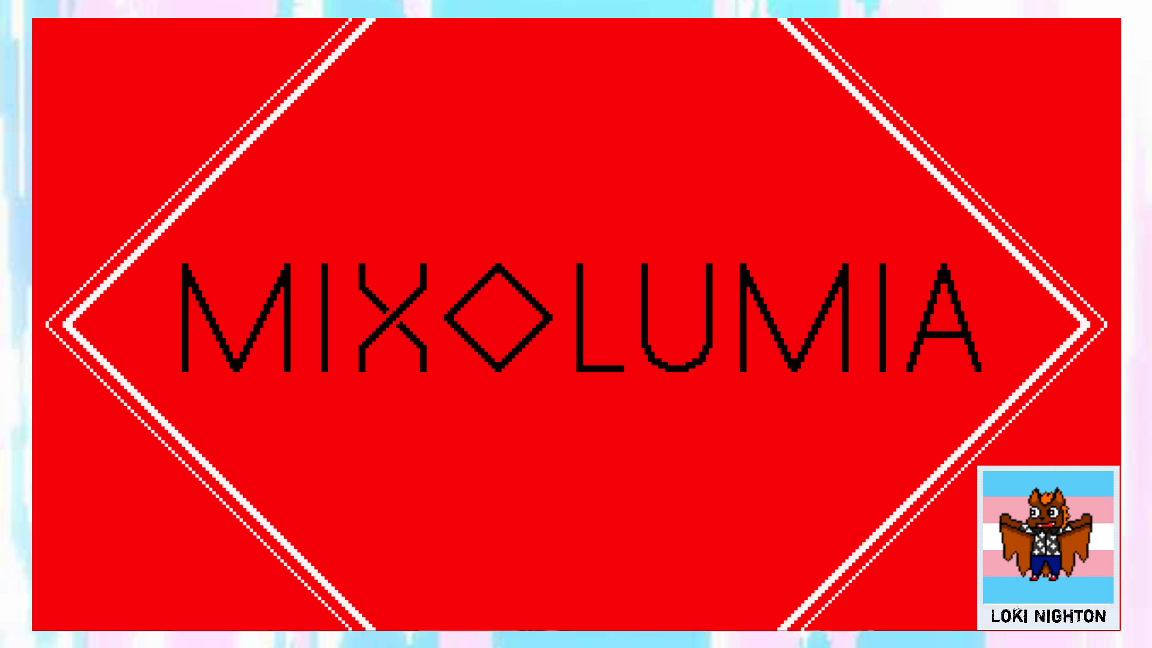[REPLACEME]
name=Mixer-lumia
0=f40009
1=ffffff
2=000000
3=b8b8b8
4=5e071a
5=567e44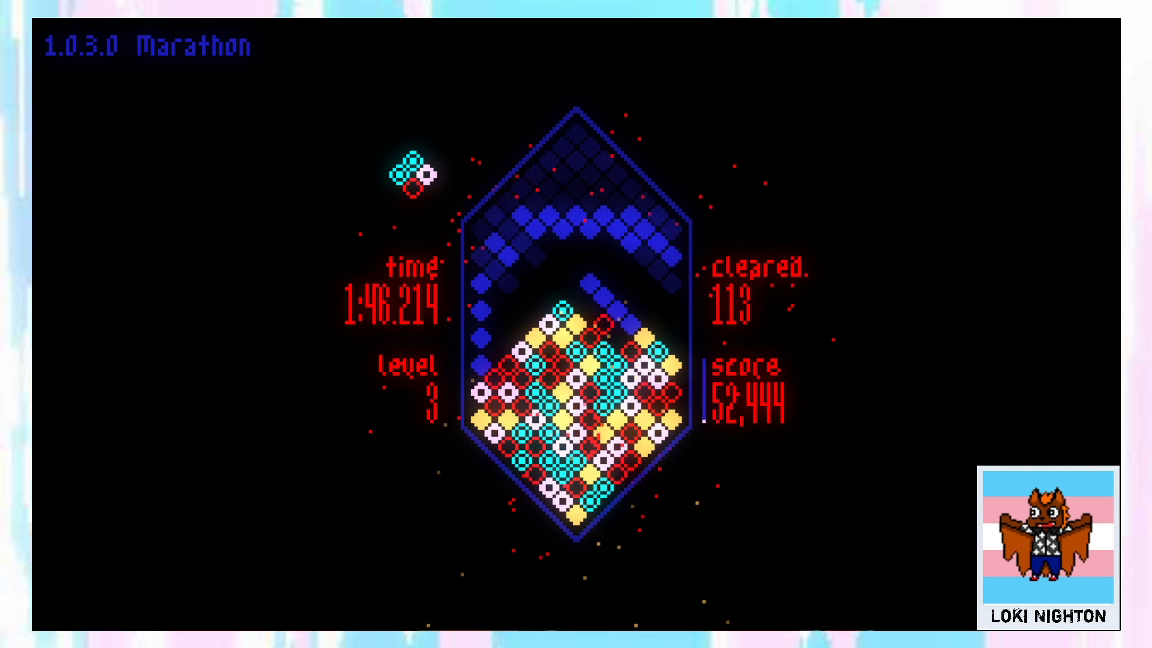[REPLACEME]
name=WAKAWAKAWAKA
0=000000
1=1919a6
2=ff0000
3=ffb852
4=ffb8ff
5=00ffff

Threw together a load of gaming inspired colour palettes (I'm especially proud of WAKAWAKAWAKA) as well as a soft drink inspired 'Mixer-lumia' palette ^w^

Just played a couple rounds with Ash Heap -- loving it!!

Since I'm working on a review, I decided to make a thheme based on the green colors of my YouTube channel. Far from perfect, because, well, I'm not an artist, but good enough for my needs to show how it's modular.

```[REPLACEME]
name=Tsundoku Zone
0=d0e0d3
1=ffffff
2=74aa7c
3=498c57
4=74aa7c
5=a5c3ac```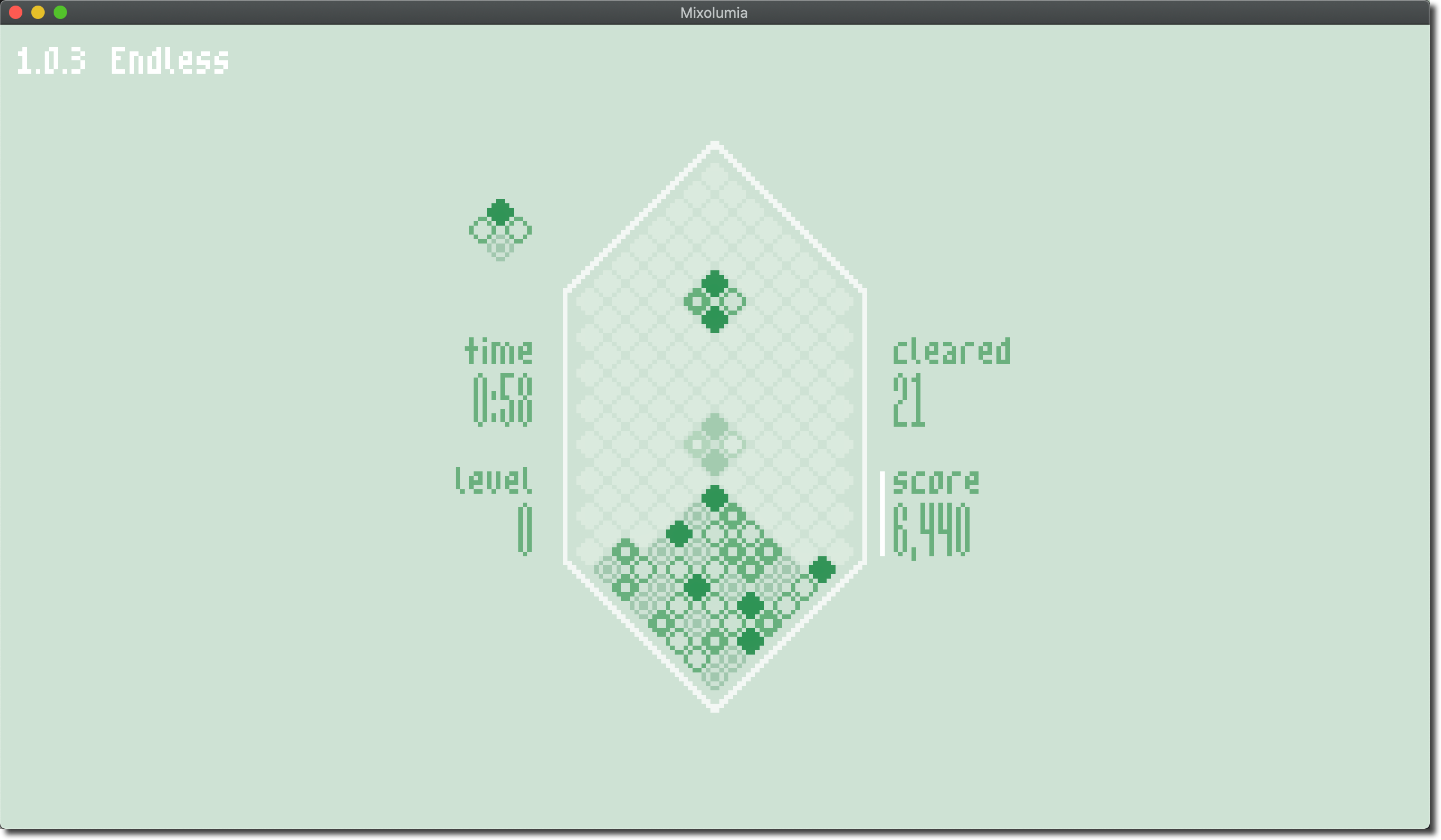Notably, on Mac, I've found it easiest to just locate the game, Open Package Contents, and find the pallettes.ini file to edit.

Developer

Nice! Be sure to back up your custom colors if you're editing the file in the app package, it may get overwritten on update.

(2 edits) (+2)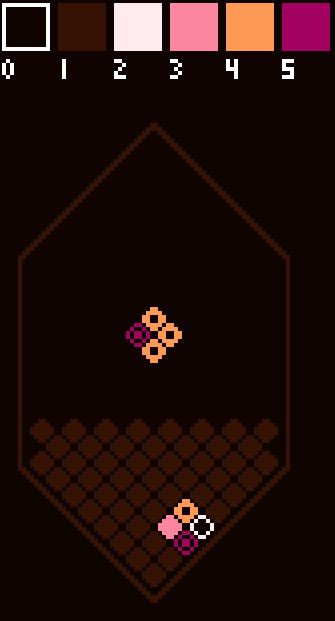I am a big ol' lesbian so i made a dark lesbian flag theme!

name=Lesbian Rights!
author=Autumn
0=0f0401
1=361204
2=ffebef
3=fc86a0
4=fd9855
5=a20161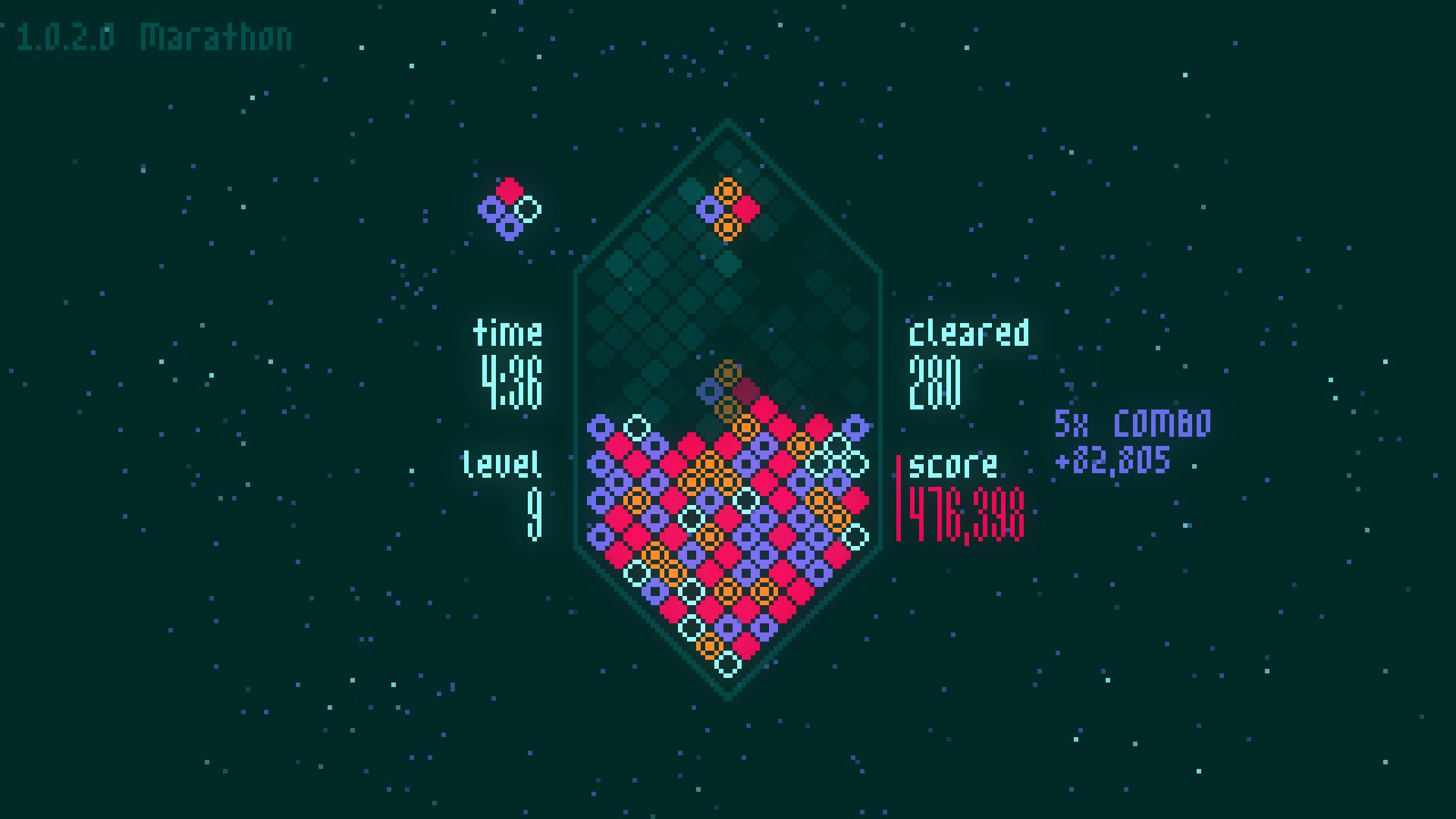Here's mine. <3

name="Lovely"
author="@thatsmytrunks"
0="00201e"
1="024442"
2="8ee8e6"
3="d30548"
4="6361d6"
5="f37c1c"

Lovely, and this makes me wish there was a star field zoom going on all the time in the background of the game! @davemakes consider this an official suggestion for warp mode

Maybe you would like to visit Santa Barbara someday?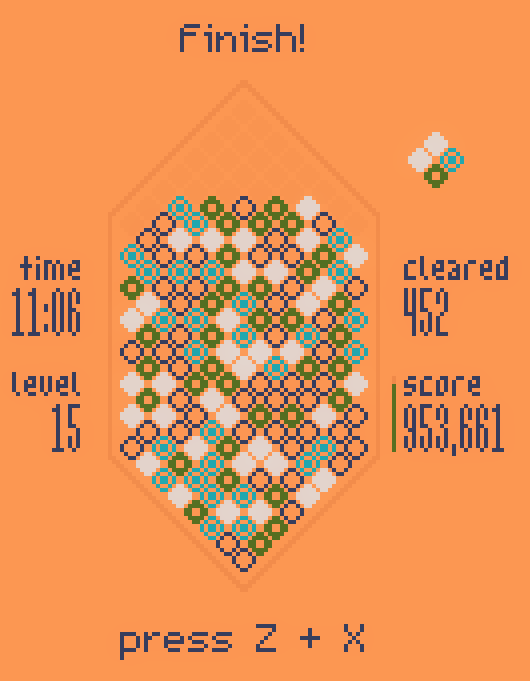```name=Santa Barbara
author=Jeri Mason @jericmason
0=fc9653
1=ee884a
2=2e3a5f
3=e2d3ce
4=4f6d20
5=1aa6b1```
Developer(+1)

ohhhh it's like a mango smoothie!

Fans of the board game Azul may recognize this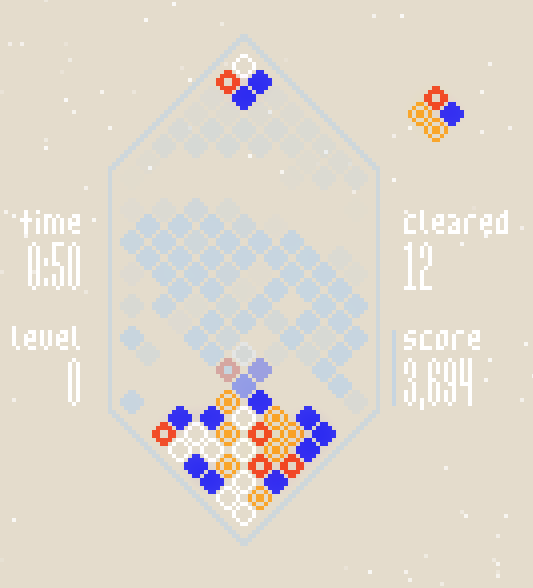``````name=Azulite
author=Jeri Mason @jericmason
0=e2d9c8
1=c2d2dd
2=ffffff
3=2626f0
4=f0401a
5=f89e1c
``````
(+1)

Wanted to experiment with palettes & try to make something based on my Vim color scheme. Definitely a bit bright for nighttime, but at least it's very pink...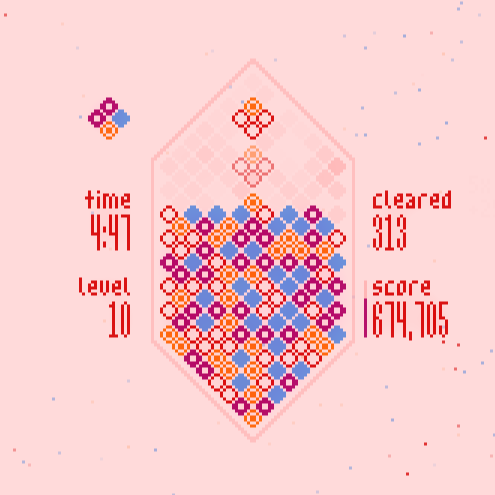`name="decolletage"author="@brhfl"0=ffd7d71=ffafaf2=d700003=5f87d74=af005f5=ff5f00`

A palette inspired by Yume Nikki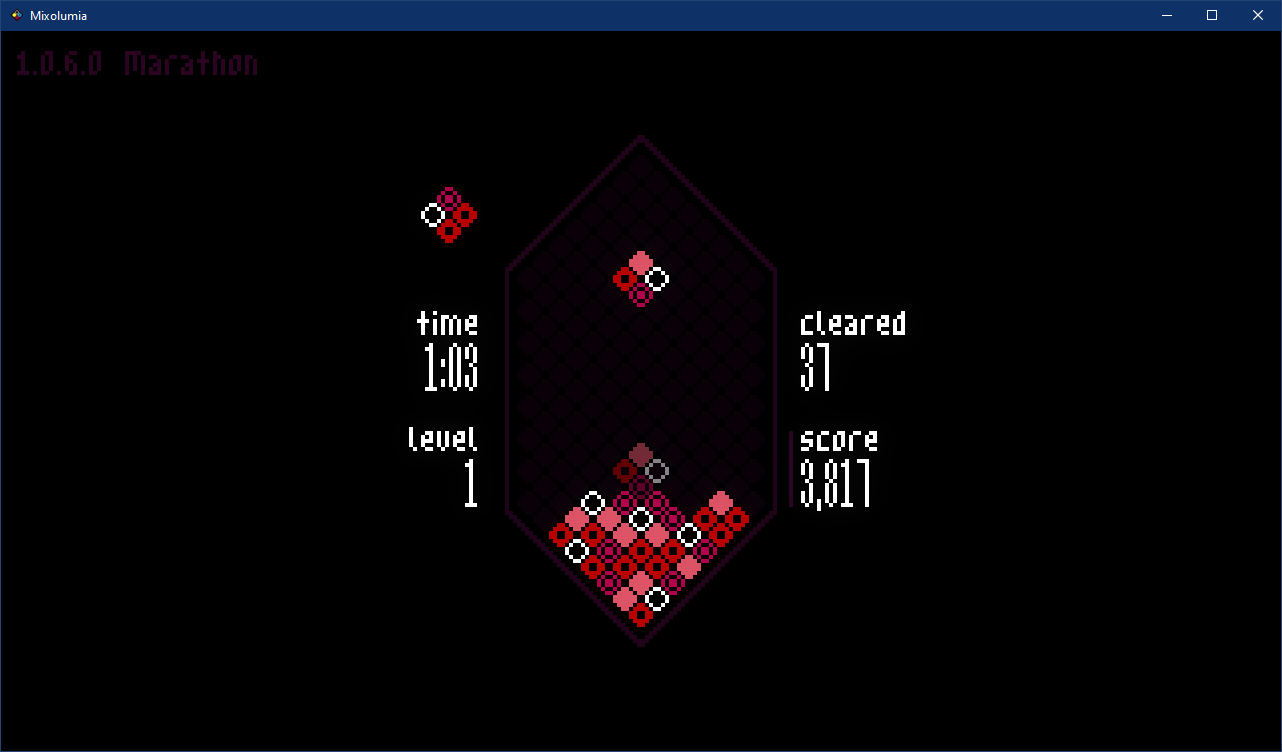```name="Madotsuki"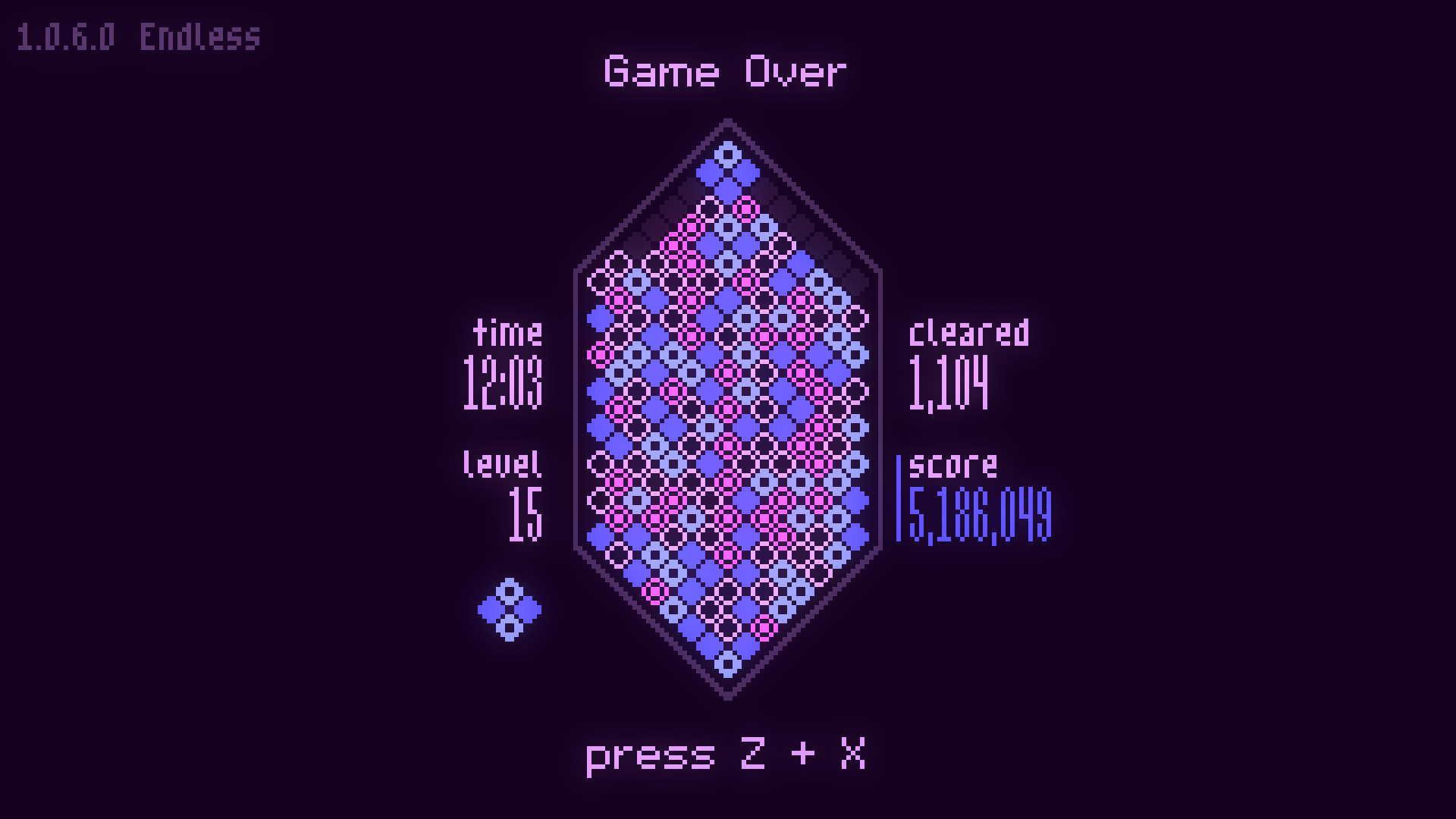`name="D_W-Custom-02"author="@dwoboyle"0=1000181=5034612=d496ff3=554fff4=8790ff5=f255fd`